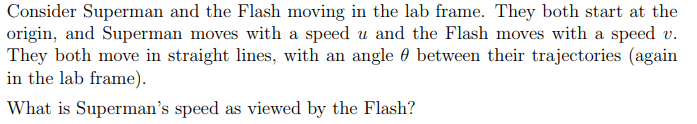# Speed of a moving object, according to another moving object

## Homework Statement

Special Relativity Question.

Consider objects 1 and 2 moving in the lab frame; they both start at the origin, and #1 moves with a speed u and #2 moves with a speed v. They both move in straight lines, with an angle θ between their trajectories (again in the lab frame). What is #1's speed as viewed by #2?## Homework Equations

x' = γ(x-vt)
t' = γ(t-(vx/c2))
u' = (u±v)/(1±(uv/c2))
Length Contractions
Lorentz Transformations
[/B]
Where γ = 1/((1-(v2/c2))),
v is the velocity of the frame S' relative to S,
and u' is the velocity of some object in the new frame S'.
(These are general equations, not variables from the question).

## The Attempt at a Solution

So I assumed the lab frame (I'll call it S) was at rest, and I had #2's (Flash) velocity along the x-axis, and #1's (Superman) velocity at some angle θ1 above that, both starting from the origin. To simplify things, I made another frame S' where the new Vframe=v, so in this new frame object 2's speed is v' = 0, and u' is what we're looking for I think.

In the j direction, nothing would change in S' for object 1, and the velocity in the i direction would be less so the angle would change, as well as the magnitude from that, so we now have θ2. However, I'm not really sure how I would use this new angle. From using the u' equation I have above, I got:

u'x = (u*cos(θ1) - v) / (1 - (u*cos(θ1)*v / (c2)))

(Would this be 1+ uc/c^2 or 1- uv/c^2 in the denominator? I'm not sure how to tell)

u'y = (u*sin(θ1) - 0) / (1 - 0)

I got 1 - 0 because the V of the frame in the j direction is 0

However none of that really took into account θ2, and I'm not sure how to get my final answer with the x and y components. With relativity can I just do the Pythagorean theorem as normal?

#### Attachments

Orodruin
Staff Emeritus
Homework Helper
Gold Member
In the j direction, nothing would change in S' for object 1,

From using the u' equation I have above, I got:

u'x = (u*cos(θ1) - v) / (1 - (u*cos(θ1)*v / (c2)))
This is not correct either. You cannot treat the components separately. You need to Lorentz transform the worldline of 1 to S’ and identify tge corresponding velocity. Alternatively you can use 4-vectors if you are familiar with them.

•Phantoful
Nathanael
Homework Helper
u'x = (u*cos(θ1) - v) / (1 - (u*cos(θ1)*v / (c2)))

This is not correct either.
I’m hesitant to go against you, but I’m pretty sure that part is correct, isn’t it?

Anyway @Phantoful the y component is definitely not the same when changing frames. (A boost along the x-direction leave y-displacements unchanged, not y-velocities.)

Try considering two events located on superman that are Δt apart in the lab frame. What is Δx and Δy in the lab frame? What is Δt’, Δx’, Δy’ between these events in Flash’s frame?

•Phantoful
Orodruin
Staff Emeritus
Yes, you are correct. I blame lack of sleep last night.Don't hesitate to point out when I screw up.
•